Function Repository Resource:

# DiagonalizeMatrix

Get the diagonalized matrix of a given matrix

Contributed by: Wolfram|Alpha Math Team
 ResourceFunction["DiagonalizeMatrix"][mat] returns the diagonalized matrix for the matrix mat.

## Examples

### Basic Examples (1)

Return the diagonalized matrix for a matrix:

 In:=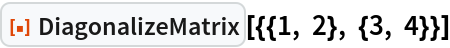Out=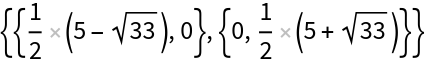In:=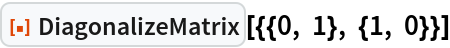Out=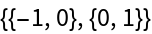### Scope (2)

DiagonalizeMatrix works with complex-valued matrices:

 In:=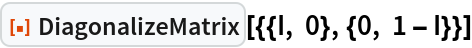Out=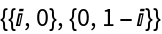DiagonalizeMatrix works with matrices containing symbolic elements:

 In:=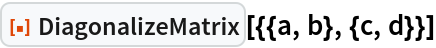Out=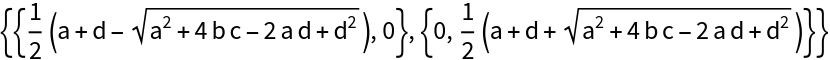### Properties and Relations (2)

When the matrix is diagonalizable, DiagonalizeMatrix returns the diagonal matrix from JordanDecomposition:

 In:=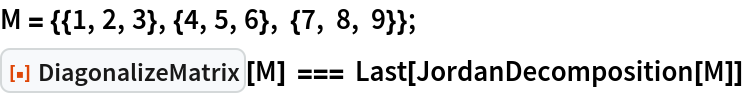Out=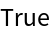The Eigenvalues of the matrix appear along the diagonal of DiagonalizeMatrix:

 In:=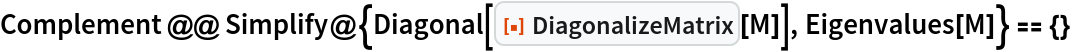Out=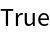### Possible Issues (2)

The function returns unevaluated when the matrix is not square:

 In:=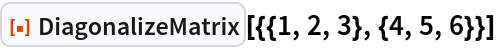Out=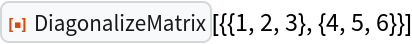The function returns unevaluated when the matrix is not diagonalizable:

 In:=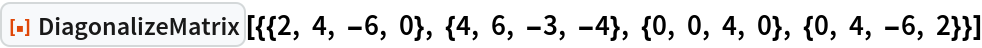Out=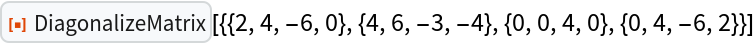For non-diagonalizable square matrices, a form that is "almost" diagonalized exists, having zeros and ones on the superdiagonal and zeros elsewhere than the main diagonal. It can be found using JordanDecomposition:

 In:=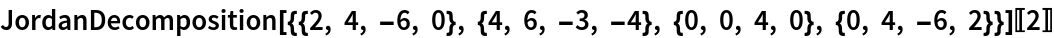Out=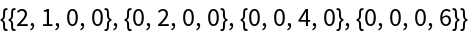## Publisher

Wolfram|Alpha Math Team

## Version History

• 3.0.0 – 23 March 2023
• 2.0.1 – 02 February 2021
• 2.0.0 – 02 October 2020
• 1.0.0 – 24 March 2020